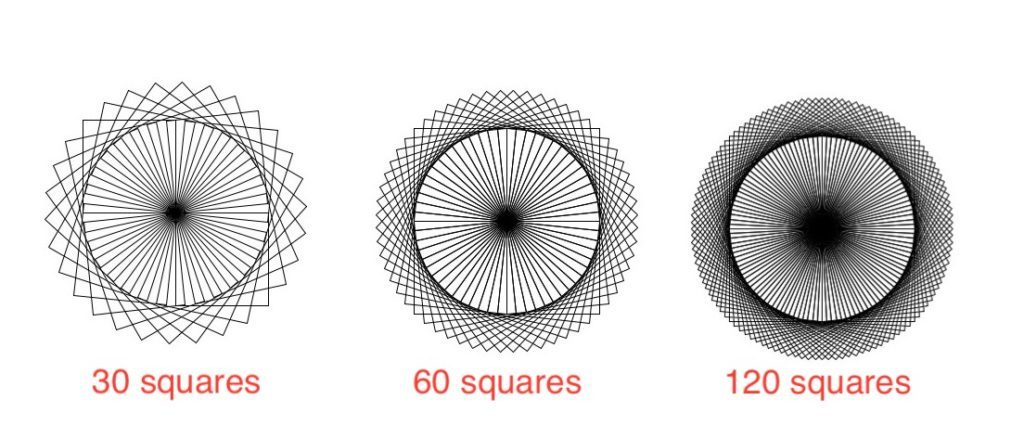Open in App
Not now

# Circle of Squares using Python

• Last Updated : 05 Jun, 2020

Turtle library enables users to draw pictures or shapes using commands, providing them with a virtual canvas. turtle comes with Python’s Standard Library. It needs a version of Python with Tk support, as it uses tkinter for the graphics. In this article, we will generate a circular pattern out of squares using Python’s turtle module.

Examples:Pattern for different number of squares

Approach:

For drawing the circle, we need to draw n number of square, each time rotating the turtle cursor by d degrees. n and d are chosen such that `n*d=360`, so as to complete a full circle. In the implementation below we will draw a circle of 60 squares, rotating the cursor each time by 6 degrees.

Functions used:

• fd(x) : draw the cursor forward by x pixels.
• rt(x) : rotates the facing direction of the cursor by x degrees to the right.

Below is the implementation.

 `from` `turtle ``import` `*`` ` ` ` `# loop for number of squares``for` `i ``in` `range``(``60``):``     ` `    ``# loop for drawing each square``    ``for` `j ``in` `range``(``4``):``         ` `        ``# drawing each side of``        ``# square of length 100 ``        ``fd(``100``)``         ` `        ``# turning 90 degrees``        ``# to the right``        ``rt(``90``)``         ` `    ``# turning 6 degrees for``    ``# the next square``    ``rt(``6``)`

Output:

My Personal Notes arrow_drop_up